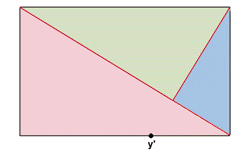INSIGHT IMAGING Thoughts and Images

# THE GOLDEN MEANLet’s start with an introduction of a technique that is well known for many centuries now: The “Golden Mean” (sometimes called “Golden Section”) is a geometric formula by the ancient Greeks. A composition following this rule is thought to be “harmonious”. The principal idea behind it is to provide geometric lines which can be traversed when viewing a composition. The Golden Mean was a major guideline for many artists/painters so it is certainly worth to have in mind for modern day photographers as well.

### Theory – Part I

Well, let’s begin with some words about the theory. The formula starts with a perfect square (marked blue in illustration A). Now we divide the base of the square into two equal parts. We take point x as the middle of a circle with a radius of the distance between point x and y. Thereafter we expand the base of the square till it hits the circle at point z. Now the square can be transformed to a rectangle with a proportion ratio of 5:8. The ratio of A to C is the same as the one from A to B. Luckily the 5:8 ration fits pretty close to the ratio of the 35mm format (24x36mm = 5:7.5).Illustration A

### Theory – Part II

So now we’ve something which is thought to be a “perfect” rectangle. What’s next ? We draw a line from the upper left to the lower right edge of the rectangle (see illustration B) and another line from the upper right directed towards point y’ (taken from illustration A) till it hits the first cross line. Obviously this divides the rectangle into three different sections.
In principal we’re finished with the “Golden Mean” now. Just try to find objects/parts in your scene that fit roughly into these three sections and … you have a “harmonious” composition.
You can vary the formula by flipping and/or mirroring the schematic rectangle from illustration B.Illustration B